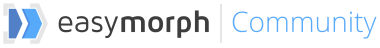# How to calculate interim and ending amounts having starting and transaction amounts

In accounting and inventory management it’s sometimes necessary to calculate the ending amount and all interim amounts after every transaction in a period using the starting balance for that period and a list of transactions happened during that period.

In EasyMorph this can be calculated using cumulative summation. However, for people coming from Excel it may be not clear why use cumulative summation. Here is an example that explains it:

Cumulative summation
Let’s assume we have a company that sells apples. As of January 1st, the company had 100 apples. During the year, the company had 3 transactions:

1. Customer order #1 - Sold 20 apples
2. Customer order #2 - Sold 40 apples
3. Supplier order #3 - Bought 30 apples

How many apples does the company have by the end of the year? How many apples did it have after each transaction?

If we used recursive calculation (as in Excel) then the amount of apples after each transaction could be calculated depending on the previous interim amount as follows:

``````[interim amount 1] = [Starting balance] - [Order #1]
[interim amount 2] = [interim amount 1] - [Order #2]
[interim amount 3] = [interim amount 2] - [Order #3] = [Ending amount]
``````

However EasyMorph works in a different way than Excel, and it’s not possible in EasyMorph create recursive calculations as in Excel. What do we do in this case?

If we look closer, the calculation above can be rewritten by substituting interim amounts so that they don’t depend on each other:

``````[interim amount 1] = [Starting balance] - [Order #1]
[interim amount 2] = [Starting balance] - [Order #1] - [Order #2]
[interim amount 3] = [Starting balance] - [Order #1] - [Order #2] - [Order #3] = [Ending amount]
``````

Which is, effectively:

``````[interim amount 1] = [Starting balance] - [Order #1]
[interim amount 2] = [Starting balance] - ([Order #1] + [Order #2])
[interim amount 3] = [Starting balance] - ([Order #1] + [Order #2] + [Order #3]) = [Ending amount]
``````

Now in order to calculate each interim amount only the starting amount and the cumulative sum of each order (transaction) are necessary.

Multiple products
Using cumulative summation it’s possible to calculate interim amounts for transactions that involve different products.

Below is a sample EasyMorph project where interim amounts are calculated for transactions with apples and bananas:

interim-amounts.morph (6.3 KB)
Book1.xlsx (9.8 KB)